# The Shift Register

## The Shift Register

The Shift Register is a sequential logic circuit which is used for the storage or the transfer of data in the form of binary numbers.

This sequential device loads the data present on its inputs and then moves or “shifts” it to its output once every clock cycle, hence the name “shift register”.

A shift register basically consists of several single bit “D-Type Data Latches”, one for each data bit, connected together in a serial type daisy-chain arrangement so that the output from one data latch becomes the input of the next latch and so on.

Data bits may be fed in or out of a shift register serially, that is one after the other from either the left or the right direction, or all together at the same time in a parallel configuration.

The number of individual data latches required to make up a single Shift Register device is usually determined by the number of bits to be stored.

Shift Registers are commonly used inside calculators or computers to store data such as two binary numbers before they are added together, or to convert the data from either a serial to parallel or parallel to serial format.

The individual data latches that make up a single shift register are all driven by a common clock ( Clk ) signal making them synchronous devices.

Shift register IC’s are generally provided with a clear or reset connection so that they can be “SET” or “RESET” as required.

Generally, shift registers operate in one of four different modes with the basic movement of data through a shift register such as:

1. Serial-in to Serial-out (SISO) The register is loaded with serial data,one bit at a time, and shifted serially out of the register, one bit at a time in either a left or right direction under clock control.
2. Serial-in to Parallel-out (SIPO):  The register is loaded with serial data, one bit at a time, with the stored data being available at the output in parallel form.
3. Parallel-in to Serial-out (PISO): The parallel data is loaded into the register simultaneously and is shifted out of the register serially one bit at a time under clock control.
4. Parallel-in to Parallel-out (PIPO) The parallel data is loaded simultaneously into the register, and transferred together to their respective outputs by the same clock pulse.

The effect of data movement from left to right through a shift register can be presented graphically as: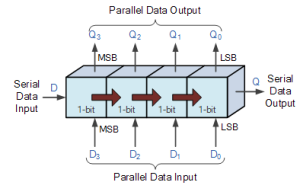Fig.1

### Serial-in to Serial-out (SISO) Shift Register

Let all the flip-flop be initially in the reset condition i.e. QA = QB = QC = QD = 0.

We enter  a four bit binary number 1 1 1 1 into the register.

This number should be applied to Din bit, with the LSB bit applied first. The D input of FFA i.e. DA is connected to serial data input Din. Output of FFA i.e. QA is connected to the input of the next flip-flop i.e. DB and so on.

#### Block Diagram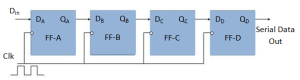Fig.2

#### Operation

Before the application of clock signal let all the flip-flop be initially in the reset condition i.e. QA = QB = QC = QD = 0 and apply  the LSB bit of the number to be entered to Din. So Din=DA=1.

Now apply the clock.

On the first falling edge of clock, the FFA is set, and stored word in the register is,

QA QB QC QD = 1000.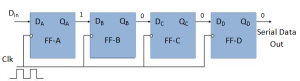Fig.3

Apply the next bit to Din. So Din=1.

As soon as the next negative edge of the clock hits, FF-B will set and the stored word change to

QA QB QC QD = 1100.Fig.4

Apply the next bit to be stored i.e. 1 to Din.

Apply the clock pulse. As soon as the third negative clock edge hits, FF-C will be set and output will be modified to QA QB QC QD = 1110.Fig.5

Similarly with Din=1 and with the fourth negative clock edge arriving, the stored word in the register is QA QB QC QD = 1111.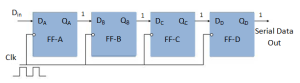Fig.6

#### Truth Table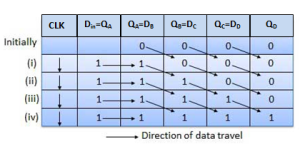#### Wave Forms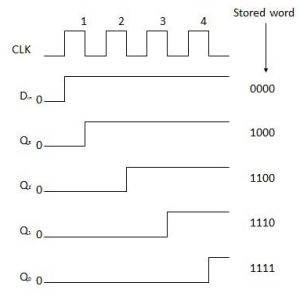Fig.7

### Serial-in to Parallel-out Shift Register (SIPO)

• In such types of operations, the data is entered serially and taken out in parallel fashion.
• Data is loaded bit by bit. The outputs are disabled as long as the data is loading.
• As soon as the data loading gets completed, all the flip-flops contain their required data, the outputs are enabled so that all the loaded data is made available over all the output lines at the same time.
• 4 clock cycles are required to load a four bit word. Hence the speed of operation of SIPO mode is same as that of SISO mode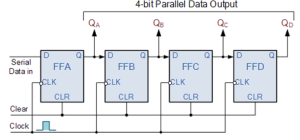Fig.8

#### Parallel Input Serial Output (PISO)

In this type of shift register data bits are entered in parallel fashion.

The circuit shown below is a four bit parallel input serial output  shift register.

We can note that output of previous Flip Flop is connected to the input of the next one via a combinational circuit.

The binary input word B0,B1,B2,B3 is applied though the same combinational circuit.

There are two modes in which this circuit can work namely shift mode or load mode.

When the shift/load bar line is low i.e. logic “0”, the AND gate 2,4 and 6 become active.

They will pass B1,B2,B3bits to the corresponding flip-flops.

On the low going edge of clock, the binary input B0,B1,B2,B3 will get loaded into the corresponding flip-flops. Thus parallel loading takes place.

#### SHIFT MODE

When the shift/load bar line is high i.e logic “1”, the AND gate 2,4 and 6 become inactive.

But the AND gate 1,3 and 5 become active.

Therefore the shifting of data takes place from left to right bit by bit on application of clock pulses. Thus the parallel in serial out operation take place.

#### Block Diagram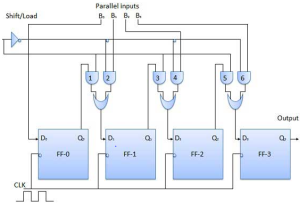Fig.9

#### Parallel Input Parallel Output (PIPO)

In this mode, the 4 bit binary input B0,B1,B2,B3 is applied to the data inputs D0,D1,D2,D3 respectively of the four flip-flops.

As soon as a negative clock edge is applied, the input binary bits will be loaded into the flip-flops simultaneously.

The loaded bits will appear simultaneously to the output side.

Only clock pulse is essential to load all the bits.

#### Block Diagram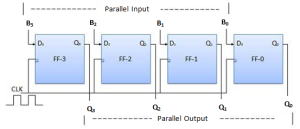Fig.10

### Bidirectional Shift Register

• If a binary number is shifted left by one position then it is equivalent to multiplying the original number by 2. Similarly if a binary number is shifted right by one position then it is equivalent to dividing the original number by 2.
• Hence if we want to use the shift register to multiply and divide the given binary number, then we should be able to move the data in either left or right direction.
• Such a register is called as a bi-directional register.
• A four bit bi-directional shift register is shown in fig.11
• There are two serial inputs namely the serial right shift data input DR  and the serial left shift data input DL along with a mode select input (M).

#### Block Diagram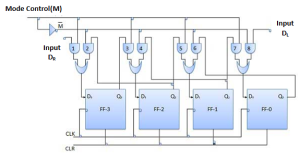Fig.11

#### With M = 1 : Shift right operation:

• If M = 1, then the AND gates 1,3,5 and 7 are enable whereas the remaining AND gates 2,4,6 and 8 will be disabled.
• The data at DR is shifted to right bit by bit from FF-3 to FF-0 on the application of clock pulses.
• Thus with M = 1 we get the serial right shift operation.

#### With M = 0 : Shift left operation:

• When the mode control M is connected to 0 then the AND gates 2,4,6 and 8 are enabled while 1,3,5 and 7 are disabled.
• The data at DL is shifted left bit by bit from FF-0 to FF-3 on the application of clock pulses.
• Thus with M = 0 we get the serial right shift operation.

#### Universal Shift Register

A  shift register which can shift the data in both directions as well as load it parallely, is known as a universal shift register. The shift register is capable of performing the following operation

• Left shifting
• Right shifting

The mode control input is connected to logic 1 for parallel loading operation whereas it is connected to 0 for serial shifting.

With mode control pin connected to ground, the universal shift register acts as a bi-directional register.

For serial left operation, the input is applied to the serial input which goes to AND gate-1 shown in figure.12. Whereas for the shift right operation, the serial input is applied to D input.

#### BLOCK DIAGRAM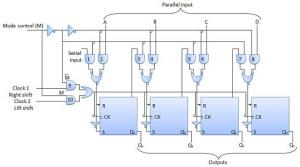Fig.12